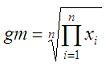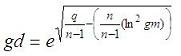# Geometric mean and geometric deviation

This program computes the geometric mean and geometric deviation of a set of data. We obtain the results in two ways, using iterations and available vectorized operations in Matlab.

The geom. mean is given by this formulathis means that it is the nth-root of the product of all the elements being considered. n is the number of elements in the set.

The geom. deviation is given by this formulawhere q is a sum including the natural logarithms of the elements in the set.We can use iterations to calculate what we need. In Matlab, this is not the most efficient way to do it, but we can implement the same algorithm in other languages...

function [gm, gd] = geo_mean_dev(x)
% Take into account the number of elements in the vector
n = length(x);

% Initialize some variables
gm = 1;
q = 0;

% Iterate through all of the elements
for i = 1 : n
d = x(i);

% Compute mean
gm = gm * d^(1/n);

% Accumulate intermediate term for deviation
q = q + log(d)^2;
end

% Compute deviation
gd = abs(exp(sqrt(q/(n-1)-(n/(n-1)*(log(gm))^2))));

We can test our function as follows (from the command window or from another script):

x = [3 5 8 3 7 2];
[gm1, gd1]= geo_mean_dev(x)

Matlab response is

gm1 =     4.1407
gd1 =     1.7237

We can also use the vectorized form to make it easier and faster...
(note that the 'log' function performs the natural logarithm of a number, while the 'log10' function performs the log in base 10)

n = length(x);

% The 'prod' function gets the multiplication of all the elements

gm2 = prod(x)^(1/n)

% The 'sum' function gets the sum of the vector

q = sum(log(x).^2);

% and now we can imlement the last formula
gd2 = exp(sqrt(q/(n-1)-(n/(n-1)*log(gm2)^2)))

Again, Matlab response is

gm2 =     4.1407
gd2 =     1.7237

From 'Geometric Mean' to home

From 'Geometric Mean' to 'Matlab Cookbook'